Junction Diodes

# Junction Diodes Notes | Study Electronic Devices - Electronics and Communication Engineering (ECE)

## Document Description: Junction Diodes for Electronics and Communication Engineering (ECE) 2022 is part of Electronic Devices preparation. The notes and questions for Junction Diodes have been prepared according to the Electronics and Communication Engineering (ECE) exam syllabus. Information about Junction Diodes covers topics like and Junction Diodes Example, for Electronics and Communication Engineering (ECE) 2022 Exam. Find important definitions, questions, notes, meanings, examples, exercises and tests below for Junction Diodes.

Introduction of Junction Diodes in English is available as part of our Electronic Devices for Electronics and Communication Engineering (ECE) & Junction Diodes in Hindi for Electronic Devices course. Download more important topics related with notes, lectures and mock test series for Electronics and Communication Engineering (ECE) Exam by signing up for free. Electronics and Communication Engineering (ECE): Junction Diodes Notes | Study Electronic Devices - Electronics and Communication Engineering (ECE)
 1 Crore+ students have signed up on EduRev. Have you?

Semiconductor Diode (p-n junction diode)

Majority carrier electrons in the n-region will begin diffusing into the p-region and majority carrier holes in the p-region will be diffusing into the n-region. If we assume there are no external excitation to the semiconductor, then this diffusion process cannot continue indefinitely. As electrons diffuse from the n-region, positively charged donor atoms are left behind. Similarly, as holes diffuse from the p-region, they uncover negatively charged acceptor atoms. The un-neutralized ions in the neighbourhood of the junction are referred to as uncovered charges. The general shape of the charge density ‘ρ’ depends upon how the diode is doped. Since the region of the junction is depleted of mobile charges, it is called depletion region, the space-charge region, or the transition region.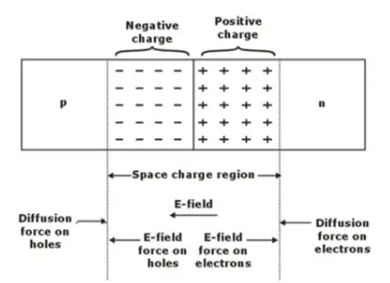The net positive and negative charges in ‘n’ and ‘p’ regions induce an electric fields in the region near the metallurgical junction, in the direction from the positive to the negative charge, or from the n to the p region.
Density gradients still exist in the exist in the majority carrier concentrations at each edge of the space charge region and producing a “diffusion force” that acts on the majority carriers as shown in Figure 1. The electric field in the space charge region produces another force on the electrons and holes which in the opposite direction to the diffusion force for each type of particle. In thermal equilibrium, the diffusion force and the field force exactly balance each other.

I-V Characteristics of a p-n Junction Diode

Below figure indicates the characteristic curve consisting of three distinct regions: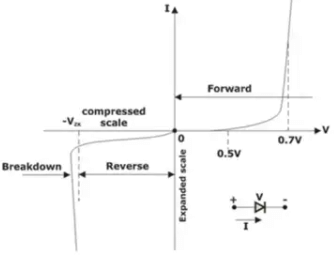p-n Junction characteristic Note: When PN junction is reverse biased, the reverse voltage must be always less than breakdown voltage of device, otherwise the normal diode will be damaged.

Contact Potential or Built-in potential

Let the PN junction is kept either open circuit condition or unbiased condition.
Mathematically,
V0 = Vbi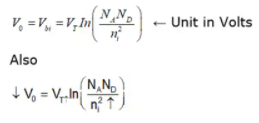Note

• Contact Potential, V0 is a function of temperature.
• Contact Potential, V0 decreases with the temperature.
• For 1ºC rise in temperature, V0 decreases by 2.5 mV.

Biasing of a Diode

The electric field across the junction has a fixed polarity called barrier potential or height of the barrier. A popular semiconductor device is formed using a p-n junction called p-n junction diode.

No Applied Bias (VD = 0V): In the absence of an applied bias voltage, the net flow of charge in anyone direction for a semiconductor diode is zero.

Forward Bias (VD > 0V):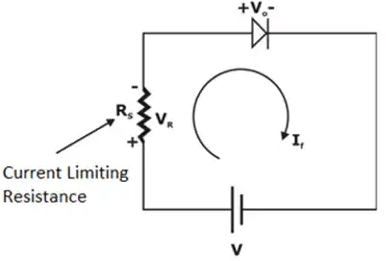Rs = Current Limiting Resistance
VD = Forward Voltage across diode
By KVL we get:
V = VR + VD
V = If Rs + If Rf
Rf = Forward resistance of diode
The General formula of forward current, if (diode current) is: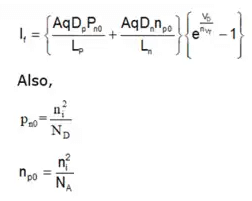So,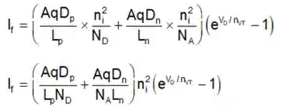AqDp/LpN= If due to flow of holes from P side to N side
AqDn/NALn = If due to flow of electrons from N side to P side
Also,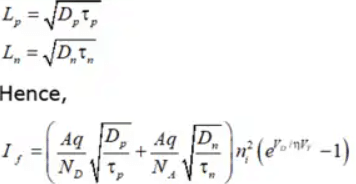The reverse saturation current in the Forward bias diode i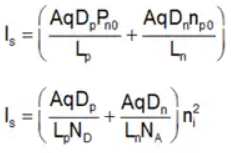AqDp/LpN= If due to flow of holes from P side to N side
AqDn/LnNA = If due to flow of electrons from N side to P side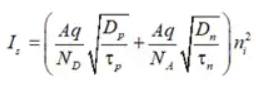A = Cross Sectional Area of Junction

Reverse Bias (VD < 0V):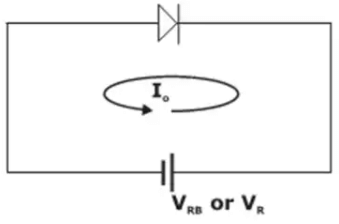When PN junction is reverse biased, the width of the depletion layer increases.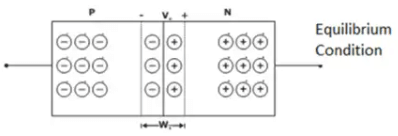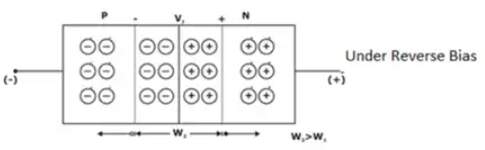Note: When PN junction is reverse biased the majority carries of P & N region will move away from the junction and this increases the region of IMMOBILE CHARGES i.e. the width of the depletion layer is increased.

Reverse Bias Breakdown in Diode

We have found that a p-n junction biased in the reverse direction exhibits a small, essentially voltage-independent saturation current. This is true until a critical reverse bias is reached, for which reverse breakdown occurs [Fig. (18)]. At this critical voltage (VBr) the reverse current through the diode increases sharply, and relatively large currents can flow with little further increase in voltage. The existence of a critical breakdown voltage introduces almost a right-angle appearance to the reverse characteristic of most diodes.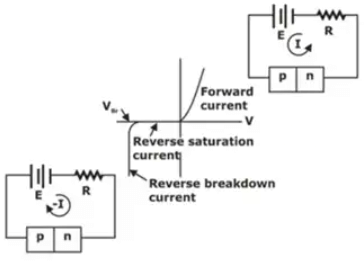Reverse breakdown in a p-n Junction

Static and Dynamic Resistance

The static resistance of a diode is defined as the ratio V/I of the voltage to the current. At any point on the volt-ampere characteristic of the diode the resistance Rf is equal to the reciprocal of the scope of a line joining the operating point to the origin.
For small-signal operation the dynamic, or incremental, resistance r is defined as the reciprocal of the slope of the volt-ampere characteristic. RºdV/dI. For a semiconductor diode, we find from equation that the dynamic conductance where I is forward current.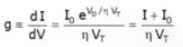for a forward bias greater than a few tenths of a volt, I >> I0 and r is given approximately by

Note

• The dynamic resistance of Ge diode with a forward current of 26 mA is 1 Ω
• Dynamic resistance in Si diode is more than in Ge diode.

Solved Example

Example 1: Find the voltage drop across each of the Silicon junction diodes shown in the figure below at room temperature. Assume that reverse saturation current flows in the circuit and the magnitude of the reverse breakdown voltage is greater than 5 Volts.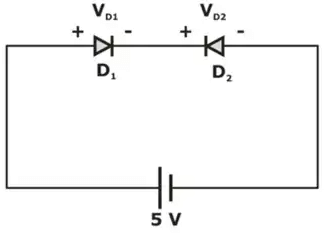Solution:
VD1 + Vd2 = 5V
VD2 = 5 -Vd1
For diode D1,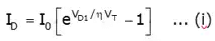For diode D2,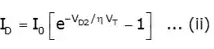from equation, (i) and (ii)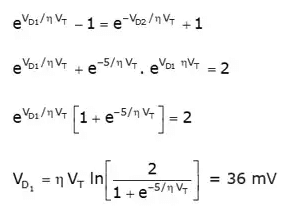VD2 = 4.964 V

Transition and Diffusion Capacitance

Transition or depletion layer capacitance:
Cr = Cj = ∈ A/W = Unit farads.
A = Cross Sectional Area Of junction
W = Width of Depletion Region
CT ∝ A
CT ∝ 1/W
For better performance of diode or BJT, the value of CT must be as small as possible.
In a reverse biased PN junction, the transition capacitance, CT
CT ∝ V-n
where
V = reverse Biased Voltage
n = constant
n = grading coefficient
n = 1/2; for Step graded diode (abrupt PN Junction diode)
= 1/3; for Linear graded diode

Diffusion Capacitance

For a forward bias p-n junction a capacitance which is much larger than the transition capacitance C, comes into picture. The origin of this larger capacitance lies in the injected charge stored near the junction outside the transition region. It is convenient to introduce an incremental capacitance defined as the rate of charge of injected charge with voltage, called the diffusion or storage capacitance CD.
C= dQ/dV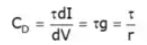where g = dl/dV the incremental conductance and r = ηVT/l. is the
incremental resistance

Therefore,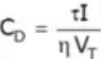Where η is a constant dependent upon semiconductor, and VT is volt equivalent temperature and τ is the mean life-time of minority carriers.

Note:

• For a reverse bias junction CD may be neglected compared with C(transition capacitance).
• For a forward bias junction CD is usually much larger than CT
• Diffusion capacitance CD is proportional to the current I.

Shockley Diodes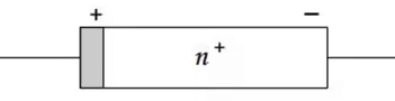• A Shockley diode is formed by bonding a metal, such as platinum, to an n-type silicon.
• This type of diodes has no depletion layer and can switch faster than ordinary diodes.
• The most important application of Shockley diodes is in digital computers
• Since a Shockley diode has a cut-in voltage of 0.25 V, they are frequently used in low-voltage rectifiers.
The document Junction Diodes Notes | Study Electronic Devices - Electronics and Communication Engineering (ECE) is a part of the Electronics and Communication Engineering (ECE) Course Electronic Devices.
All you need of Electronics and Communication Engineering (ECE) at this link: Electronics and Communication Engineering (ECE)

## Electronic Devices

10 docs|5 tests
 Use Code STAYHOME200 and get INR 200 additional OFF

## Electronic Devices

10 docs|5 tests

Track your progress, build streaks, highlight & save important lessons and more!

,

,

,

,

,

,

,

,

,

,

,

,

,

,

,

,

,

,

,

,

,

;